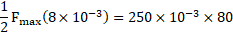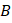# The following problemsillustrate the effect of a time–dependent force of a large average magnitude which acts on a moving object only for a short duration. Such forces are called ‘impulsive’ forces. According to the impulse-momentum theorem, impulse delivered to a body is equal to the change of linear momentum of the body A ball of mass 250 g is thrown with a speed of 30 m/s. The ball strikes a bat and it is hit straight back along the same line at a speed of 50 m/s. Variation of the interaction force, as long as the ball remains in contact with the bat, is as shown in Fig.Answer these questions Maximum force exerted by the bat on the ball is a) 2500 N b) 5000 N c) 7500 N d) 1250 N

## Question ID - 101225 :- The following problemsillustrate the effect of a time–dependent force of a large average magnitude which acts on a moving object only for a short duration. Such forces are called ‘impulsive’ forces. According to the impulse-momentum theorem, impulse delivered to a body is equal to the change of linear momentum of the body A ball of mass 250 g is thrown with a speed of 30 m/s. The ball strikes a bat and it is hit straight back along the same line at a speed of 50 m/s. Variation of the interaction force, as long as the ball remains in contact with the bat, is as shown in Fig.Answer these questions Maximum force exerted by the bat on the ball is a) 2500 N b) 5000 N c) 7500 N d) 1250 N

3537

 (b) Using area ofchange in momentumNext Question :

Two persons,of mass 60 kg andof mass 40 kg, are standing on a horizontal platform of mass 50 kg. The platform is supported on wheels on a horizontal frictionless surface and is initially at rest. Consider the following situations(i) Bothandjump from the platform simultaneously and in the same horizontal direction

(ii) A jumps first in a horizontal direction and after a few secondsalso jumps in the same direction

In both the situations above, just after the jump, the person (or) moves away from the platform with a speed of 3 m/s relative to the platform and along the horizontal Answer these questions

 In situation (i), just after bothandjump from the platform, velocity of centre of mass of the system (and the platform) is a) 2 m/s b) 6 m/s c) 5 m/s d) None of these